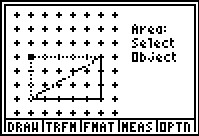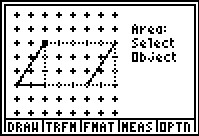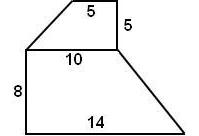# Activities

••• ##### Subject Area

• Math: Middle Grades Math: Geometry

• ##### Author6-8

45 Minutes

• ##### Device
• TI-73 Explorer™
• TI-Navigator™
• ##### Software

LearningCheck™ Creator
TI Connect™

## Dividing Up The Area#### Activity Overview

In this activity, students investigate the relationship between the area of quadrilaterals and triangles using the Geoboard application. As an extension, students will find the area of a complex figure.

#### Key Steps

•Students will use the Geoboard app to compare the areas of squares and rectangles to the area of the triangles that divide each of them. They are to determine a formula for the area of a triangle based on what they know about the formula of a rectangle.

•Then students will compare parallelograms and rectangles that have the same area to determine the relationship between the two quadrilaterals.

•As an extension, students will use what they have learned in the activity to find the area of complex figures.